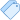July 15, 2020 Deepak Raj

### Introduction

Logistic Regression is a kind of parametric classification model. It's a binary classification machine learning algorithm. It can only be used to distinguish between 2 different categories like —

• Like if an E-mail is a spam(1) or not(0).
• Whether the tumour is malignant (1) or not (0)The theory behind Logistic Regression is very similar to the one from Linear Regression, so if you don’t know what Linear Regression is, take 5 minutes to read this Introduction.

### Types of Logistic Regression

1. Binary Logistic Regression
2. Multinomial Logistic Regression
3. Ordinal Logistic Regression

### Assumptions for Logistic Regression

1. For Logistic regression, there should be minimal or no multicollinearity among the independent variables.
2. The Logistic regression assumes that the independent variables are linearly related to the log of odds.
3. The Logistic regression usually requires a large sample size to predict properly.
4. In Logistic regression dependent, the variable should be binary.
5. The Logistic regression assumes the observations to be independent of each other.

### Iris Flower Classification


# Impoer Library for classification
import numpy as np
from sklearn.model_selection import train_test_split
from sklearn.linear_model import Logi
from sklearn.metrics import accuracy_score
from sklearn.pipeline import Pipeline
from sklearn.preprocessing import StandardScaler
import joblib


np.random.seed(10)
# split data for training and testing purpose
X = iris.data
y = iris.target
train_X, test_X, train_y, test_y = train_test_split(X, y, train_size=0.8)


# Create pipeline
pipe = Pipeline([
('scaler', StandardScaler()),
('logistic',LogisticRegression(),)
])


# fit and predict
clf = pipe.fit(train_X, train_y)
pred_y = clf.predict(test_X)
score = accuracy_score(test_y, pred_y)
print(score)


### More Articles by Author

If you like to write article or Improve this article for CodePerfectPlus mail us at codeperfectplus@gmail.com

## Popular Article##### Deploy Your First Django App With Herokutags: machine learning, programming